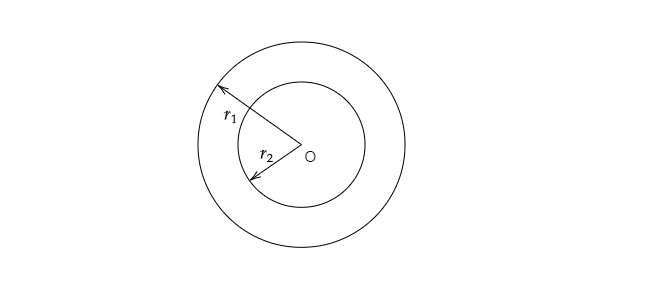# If the circumferences of two concentric circles forming a ring are $88 \ cm$ and $66 \ cm$ respectively. then find the width of the ring.

Given: Circumferences of two concentric circles forming a ring are $88 \ cm$ and $66 \ cm$ respectively.

To do: To find the width of the ring.

Solution:Let $r_1$ and $r_2$ be the radius of the given cocentric circle.

Circumference of the outer circle of the $=2\pi r_1=88$

$\Rightarrow r_1=\frac{88}{2\pi}$

$\Rightarrow r_1=\frac{88}{2\times\frac{22}{7}}$

$\Rightarrow r_1=\frac{88\times7}{2\times22}$

$\Rightarrow r_1=14\ cm$

Circumference of the inner circle of the ring$=2\pi r_2$

$\Rightarrow r_2=\frac{66}{2\pi}$

$\Rightarrow r_2=\frac{66}{2\times\frac{22}{7}}$

$\Rightarrow r_2=\frac{66\times7}{2\times22}$

$\Rightarrow r_2=\frac{21}{2}$

$\Rightarrow r_2=10.5\ cm$

Thus, the width of the ring$=r_1-r_2=14-10.5=3.5\ cm$

Updated on: 10-Oct-2022

324 Views